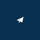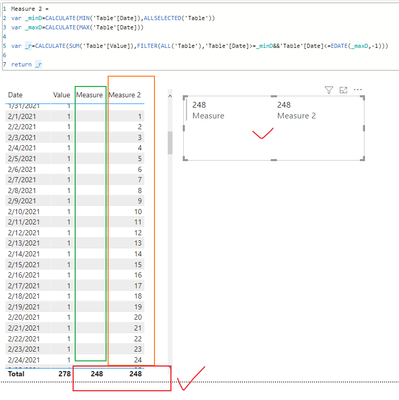cancel
Showing results for
Did you mean:

Fabric is Generally Available. Browse Fabric Presentations. Work towards your Fabric certification with the Cloud Skills Challenge.Frequent Visitor

## Compute YTD Value based on Period Selected

Hi Guys,

I just need your help in computing the YTD value based on the period selected (Start Date & End Date). Note that the value of the YTD must be minus 1 month from the End Date selected.

For example:

Period Selected: January 1, 2021 to November 15, 2021

YTD Value must show: Jan 1 to October 15. (Minus 1 month from the End Date selected)

Thank you!

1 ACCEPTED SOLUTIONMVP

Try this measure to find the YTD value:

``````Measure =
VAR _MinD =
MIN ( table[date] ) -- find the MIN date in the selected period
VAR _MaxD =
MAX ( table[date] ) -- find the MAX date in the selected period
VAR _MaxD1ML =
DATE ( YEAR ( _MaxD ), MONTH ( _MaxD ), 1 ) - 1 -- find the last date of the month before MAX date
VAR _MaxD1M =
DATE ( YEAR ( _MaxD1ML ), MONTH ( _MaxD1ML ), DAY ( _MaxD ) ) -- find the same day in the last month of the MAX date
RETURN
CALCULATE (
SUM ( table[column] ),
FILTER ( ALL ( table ), table[date] >= _MinD && table[date] <= _MaxD1M )
)``````

If this post helps, please consider accepting it as the solution to help the other members find it more quickly.

3 REPLIES 3Community Support

Hi, @greenlover

The formula @VahidDM  provided works, but be aware that you can show the correct value on the total or on the card viusal.
I have tried to make some modifications to the formula above, and you could try this formula:

``````Measure 2 =
var _minD=CALCULATE(MIN('Table'[Date]),ALLSELECTED('Table'))
var _maxD=CALCULATE(MAX('Table'[Date]))

var _r=CALCULATE(SUM('Table'[Value]),FILTER(ALL('Table'),'Table'[Date]>=_minD&&'Table'[Date]<=EDATE(_maxD,-1)))

return _r``````

Result:If you have a calendar table, then the formula @Ashish_Mathur  provided will work for you too, but note that the Edate function is followed by max(Date), like:

``````Total till previous month =
CALCULATE (
[Total],
DATESBETWEEN (
calendar[date],
MIN ( calendar[date] ),
EDATE ( MAX ( calendar[date] ), -1 )
)
)
``````

Please refer to the attachment below for details. Hope this helps.

Best Regards,
Community Support Team _ Zeon Zheng

If this post helps, then please consider Accept it as the solution to help the other members find it more quickly.Super User

Hi,

Try these measures

Total = sum(Data[Sales])

Total till previous month = calculate([Total],datesbetween(calendar[date],min(calendar[date]),edate(min(calendar[date]),-1)))

Ensure that the Date slicer is built from the Calendar Table.

Hope this helps.

Regards,
Ashish Mathur
http://www.ashishmathur.comMVP

Try this measure to find the YTD value:

``````Measure =
VAR _MinD =
MIN ( table[date] ) -- find the MIN date in the selected period
VAR _MaxD =
MAX ( table[date] ) -- find the MAX date in the selected period
VAR _MaxD1ML =
DATE ( YEAR ( _MaxD ), MONTH ( _MaxD ), 1 ) - 1 -- find the last date of the month before MAX date
VAR _MaxD1M =
DATE ( YEAR ( _MaxD1ML ), MONTH ( _MaxD1ML ), DAY ( _MaxD ) ) -- find the same day in the last month of the MAX date
RETURN
CALCULATE (
SUM ( table[column] ),
FILTER ( ALL ( table ), table[date] >= _MinD && table[date] <= _MaxD1M )
)``````

If this post helps, please consider accepting it as the solution to help the other members find it more quickly.Announcements#### Power BI Monthly Update - November 2023

Check out the November 2023 Power BI update to learn about new features.#### Fabric Community News unified experience

Read the latest Fabric Community announcements, including updates on Power BI, Synapse, Data Factory and Data Activator.#### The largest Power BI and Fabric virtual conference

130+ sessions, 130+ speakers, Product managers, MVPs, and experts. All about Power BI and Fabric. Attend online or watch the recordings.Top Solution Authors
Top Kudoed Authors
Users online (3,687)